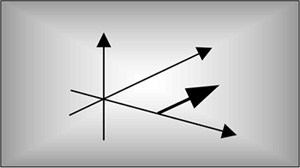# Chapter Nine. The Discrete Hilbert Transform

## Nine The Discrete Hilbert TransformThe discrete Hilbert transform is a process used to generate complex-valued signals from real-valued signals. Using complex signals in lieu of the real signals simplifies and improves the performance of many signal processing operations. If you've read about the discrete Hilbert transform in the DSP literature you've probably plowed through the mathematical descriptions of analytic functions, with the constraints on their z-transforms in their regions of convergence, and perhaps you've encountered the Cauchy integral theorem used in the definition of the Hilbert transform.[] Well, the discrete Hilbert transform is not as complicated as it first appears; this chapter attempts to support that claim.

[] The Hilbert transform is named in honor of the great German mathematician David Hilbert (1862–1943). On his tomb in Göttingen Germany is inscribed, "Wir müssen wissen, wir werden wissen." (We need to know, we shall know.)

Here we gently introduce the Hilbert transform from a practical standpoint, explain the mathematics behind its description, and show how it's used in DSP systems. In addition to providing some of the algebraic steps missing from some textbooks, we'll illustrate the time and frequency-domain characteristics of the transform, with an emphasis on the physical meaning of the quadrature (complex) signals associated with Hilbert transform applications. Finally, nonrecursive Hilbert transformer design examples and techniques for generating complex, so-called analytic signals are presented. (If you're not well versed in the notation and behavior of complex signals at this point, a review of Chapter 8 would be useful.)AmazonPrev don't be afraid of buying books Next